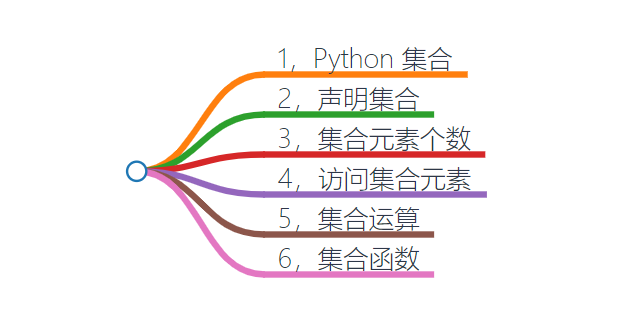# Python代码教程_Python 集合

XAMPP案例 1162浏览

—— Norm Schryer``````>>> set
<type 'set'>
``````

# 1，Python 集合

Python 中的`set` 与 `dict` 很像，唯一的不同是，`dict` 中保存的是`键值对`，而`set` 中只保存`键`，没有`值`

Python `集合`有如下特点：

• 集合中的元素是`唯一`的，`不重复`
• 集合中的元素是`无序`
• 集合中的元素可以是任意一种`不可变`类型，比如`字符串``数字``元组`
• 集合中的元素可以动态的`增加/删除`
• Python 会在需要的时候自动的`扩容``缩容`集合，方便开发者使用

# 2，声明集合

Python 集合的声明有两种方式：

• 使用`set()` 创建集合，`()` 中可为空，也可以是任意的`可迭代`类型，比如`列表``元组``字典`
• 使用大括号`{}`创建集合，从该创建方式上也能看出`集合``字典`很像

``````>>> s = set()  # 空集合
>>> s
set()
>>> s = {}     # 空的 {} 会被解析成字典
>>> s
{}
``````

``````>>> s = {1, 'abc', 1.5}       # 用 {} 创建集合
>>> s
{1, 'abc', 1.5}
>>> s = set([1, 'abc', 1.5])  # 用列表创建集合
>>> s
{1, 'abc', 1.5}
>>> s = set((1, 'abc', 1.5))  # 用元组创建集合
>>> s
{1, 'abc', 1.5}
>>> s = set({'a':1, 'b':2, 'c':3}) # 用字典创建集合
>>> s                              # 只会包含字典中的键
{'c', 'b', 'a'}
``````

``````>>> s = set([1, 2, 2, 3])  # 列表中有两个 2
>>> s                      # 集合中只有一个 2
{1, 2, 3}
``````

# 3，集合元素个数

``````>>> s = set([1, 'abc', 1.5])
>>> s
{1, 'abc', 1.5}
>>> len(s)   # 元素个数
3
``````

# 4，访问集合元素

``````>>> s = set([1, 'abc', 1.5])
>>> for i in s:
...     print(i)
...
1
abc
1.5
``````

# 5，集合运算

• `& 运算`：计算集合的`交集`
• `| 运算`：计算集合的`并集`
• `in 运算`：判断某个元素是否在集合中

``````>>> s1 = set([1, 2, 3])
>>> s2 = set([2, 3, 4])
>>> s1 & s2   # 交集
{2, 3}
>>> s1 | s2   # 并集
{1, 2, 3, 4}
``````

in 运算

``````>>> s = set([1 , 2, 3])
>>> 1 in s
True
>>> 2 not in s
False
``````

# 6，集合函数

``````>>> dir(set)
['__and__', '__class__', '__contains__',
'__delattr__', '__dir__', '__doc__',
'__eq__', '__format__', '__ge__',
'__getattribute__', '__gt__', '__hash__',
'__iand__', '__init__',
'__init_subclass__', '__ior__',
'__isub__', '__iter__', '__ixor__',
'__le__', '__len__', '__lt__', '__ne__',
'__new__', '__or__', '__rand__',
'__reduce__', '__reduce_ex__',
'__repr__', '__ror__', '__rsub__',
'__rxor__', '__setattr__', '__sizeof__',
'__str__', '__sub__', '__subclasshook__',
'__xor__',
'intersection', 'intersection_update',
'isdisjoint', 'issubset', 'issuperset',
'pop', 'remove', 'symmetric_difference',
'symmetric_difference_update', 'union',
'update']
``````

1.`add` 方法

• 添加的元素集合中不存在：只要元素类型合法，就会成功添加进去
• 添加的元素集合中已存在：不会对集合进行任何操作

``````>>> s = set([1, 3, 5]) # 初始化一个集合
>>> s
{1, 3, 5}
>>> s
{1, 3, 5, 7}
>>> s
{1, 3, 5, 7}
``````

2.`remove` 方法

``````>>> s = set([1, 3, 5])
>>> s
{1, 3, 5}
>>> s.remove(3)   # 元素 3 存在
>>> s             # 成功删除
{1, 5}
>>> s.remove(3)   # 元素 3，已不存在
Traceback (most recent call last):
File "<stdin>", line 1, in <module>
KeyError: 3       # 抛出异常
``````

3.`discard` 方法

``````>>> s = set([1, 3, 5, 6])
>>> s
{1, 5, 6}
>>> s
{1, 5, 6}
``````

4.`pop` 方法

``````>>> s = set([3, 5, 1])
>>> s.pop()  # 删除并返回 1
1
>>> s.pop()  # 删除并返回 3
3
>>> s.pop()  # 删除并返回 5
5
>>> s        # 集合为空
set()
>>> s.pop()  # 抛出异常
Traceback (most recent call last):
File "<stdin>", line 1, in <module>
KeyError: 'pop from an empty set'
``````

5.`union` 方法

``````>>> # 参数中有集合，元组，列表
>>> set.union({2, 3}, (3, 5), [5, 6])
{2, 3, 5, 6}
``````

6.`update` 方法

``````>>> s = set({2})
>>> s
{2}
>>> s.update({3, 5}, {5, 6}, [7, 8])
>>> s
{2, 3, 5, 6, 7, 8}
``````

7.`clear` 方法

``````>>> s = set([1, 3, 5])
>>> s
{1, 3, 5}
>>> s.clear()
>>> s         # 集合为空
set()
``````

8.`copy` 方法

``````>>> s = set([1, 3, 5])
>>> s
{1, 3, 5}
>>> s1 = s.copy()
>>> s1
{1, 3, 5}
``````

9.`difference` 方法

``````>>> s = set([1, 3, 5, 6])
>>> # 参数可以是任意的可迭代类型
>>> s.difference({1}, , (4, 5))
{6}
``````

10.`difference_update` 方法

``````>>> s = set([1, 3, 5, 6])
>>> s.difference_update({1}, , (4, 5))
>>> s
{6}
``````

11.`intersection` 方法

``````>>> s = set([1, 3, 5, 6])
>>> s.intersection({1}, , (4, 5)) # 相当于 s & {1} &  & (4, 5)
set()
>>> s.intersection({1, 3}, [3, 4], (3, 4, 5)) # 相当于 s & {1, 3} & [3,4] & (3, 4, 5)
{3}
``````

12.`intersection_update` 方法

``````>>> s = set([1, 3, 5, 6])
>>> s.intersection_update({1, 3}, [3,4], (3, 4, 5))
>>> s
{3}
``````

13.`isdisjoint` 方法

``````>>> s1 = set([1, 2, 3])
>>> s2 = set([3, 4, 5])
>>> s3 = set([5, 6])
>>> s1.isdisjoint(s2) # s1, s2 中有相同的元素
False
>>> s1.isdisjoint(s3) # s1, s3 中没有相同的元素
True
>>> s1.isdisjoint((4, 5)) # 参数是元组
True
``````

14.`issubset` 方法

``````>>> s = set([1, 3, 5])
>>> s.issubset({1, 3, 5, 7}) # 参数是字典
True
>>> s.issubset([1, 3, 5, 7]) # 参数是数组
True
>>> s.issubset([1, 3, 7])
False
``````

15.`issuperset` 方法

``````>>> s.issuperset({1, 3, 5, 7})
False
>>> s.issuperset({1, 3})
True
``````

16.`symmetric_difference` 方法

``````>>> s = set([1, 3, 5])
>>> s
{1, 3, 5}
>>> s.symmetric_difference([8, 9])
{1, 3, 5, 8, 9}
>>> s.symmetric_difference([8, 3])
{8, 1, 5}
``````

17.`symmetric_difference_update` 方法

``````>>> s = set([1, 3, 5])
>>> s.symmetric_difference_update({6, 8})
>>> s
{1, 3, 5, 6, 8}
>>> s.symmetric_difference_update({6, 8})
>>> s
{1, 3, 5}
``````

（完。）